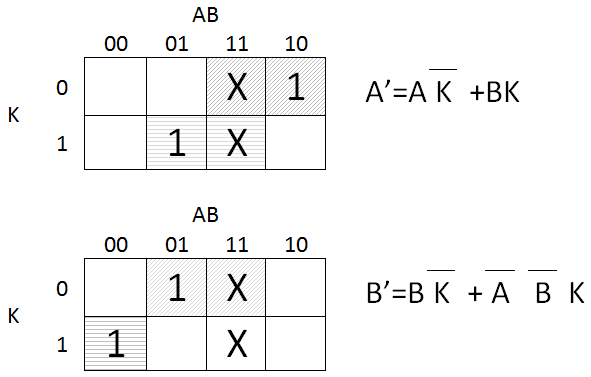# Finding a Number which Appears Once in Array where All Other Numbers Appear Three Times

by Zoran Horvat

## Problem Statement

An array of integers is given, such that each number appears exactly three times in it with exception of one number which appears only once. Write a function returning number which appears once in the array.

Example: Suppose that array consists of numbers 2, 4, 5, 1, 1, 4, 1, 2, 3, 5, 5, 4, 2. The function should return value 3, because that is the number which appears only once. All other numbers appear three times.

For similar problem, please refer to exercise Finding a Number That Appears Once in Array of Duplicated Numbers .

Another variation of the problem is covered in exercise Finding Two Numbers That Appear Once in Array of Duplicated Numbers .

## Problem Analysis

This problem resembles a similar problem where all numbers appear exactly twice, except one which appears once. Solution to this problem is explained in exercise Finding a Number That Appears Once in Array of Duplicated Numbers . Although solution is not directly applicable to problem were numbers appear three times each, we can still make use of it.

Suppose that we can isolate one particular bit in each of the numbers in the array. Now we can add values of that bit in all numbers and see whether the result is divisible by 3 or not. This test tells us about the number which appears once, because all other numbers either contribute with value 3 or with value 0. If sum is divisible with 3, then this bit in the number which appears once must be 0; otherwise it must be 1.

We can now proceed towards solving the problem by making modulo 3 sums for each of the bits in numbers in the array. But since summation modulo 3 has greatest value 2, it is obvious that intermediate results cannot be stored in one bit each. One efficient way is to keep intermediate sums in a pair of bits for each bit in the number. Alternative, we could use two integer numbers A and B, such that bits in B represent low-order bits of the modulo 3 sum, while bits in A represent high-order bits of the modulo 3 sum.

The operation then proceeds by analyzing what bitwise operations should be applied so that digits in base 3 represented by bits of A and B really resemble modulo 3 addition results. Suppose that A and B are just one bit long. In that case, when digit K (0 or 1) is added to two-bit number AB, the result of modulo 3 addition operation is given in the following table:Values X in the output indicates values that are not important because inputs can never be reached in normal operation – namely, intermediate result can never be 11 (binary), which is 3 decimal because we are performing modulo 3 addition. To extract closed form functions for new A and B, we will use Karnaugh maps .These expressions will give us the way to combine bits from all numbers in the array. Finally, each bit in the result which corresponds to AB=00 will be zero, otherwise 1. So, resulting number is just A OR B. Below is the pseudocode which implements this algorithm. Note that all Boolean operations in the code are bitwise operations.

``````function FindUniqueNumber(v, n)
-- v – array of integers
-- n – number of elements in a
begin

a = b = 0

for i = 1, n
begin
a’ = (a AND NOT v[i]) OR (b AND v[i])
b = (b AND NOT v[i]) OR (NOT a AND NOT b AND v[i])
a = a’
end

return a OR b

end
``````

Quite an astonishing piece of code when you know how complicated it is to find which number appears exactly once.

## Implementation

Below is the C# console application which lets the user enter array of integers and then prints the number which appears once in the array.

``````using System;

class Program
{

static int FindUniqueNumber(int[] array)
{

int a = 0;
int b = 0;

for (int i = 0; i < array.Length; i++)
{
int a1 = (a & ~array[i]) | (b & array[i]);
b = (b & ~array[i]) | (~a & ~b & array[i]);
a = a1;
}

return a | b;

}

static void Main()
{

while (true)
{

if (a.Length == 0)
break;

int unique = FindUniqueNumber(a);

Console.WriteLine("Number appearing once is {0}.", unique);
Console.WriteLine();

}

}

{

Console.WriteLine("Enter array (ENTER to quit):");
string[] parts = line.Split(new char[] { ' ' },
StringSplitOptions.RemoveEmptyEntries);

int[] a = new int[parts.Length];
for (int i = 0; i < a.Length; i++)
a[i] = int.Parse(parts[i]);

return a;

}
}
``````

## Demonstration

When application above is run, it produces output like this:

```            ```
Enter array (ENTER to quit):
2 4 5 1 1 4 1 2 3 5 5 4 2
Number appearing once is 3.

Enter array (ENTER to quit):
3 8 5 6 2 8 6 4 7 9 7 6 5 9 1 5 9 4 3 3 1 1 7 8 4
Number appearing once is 2.

Enter array (ENTER to quit):
```
```